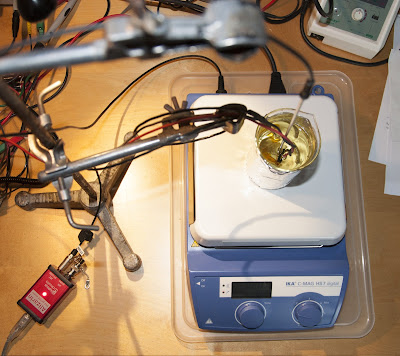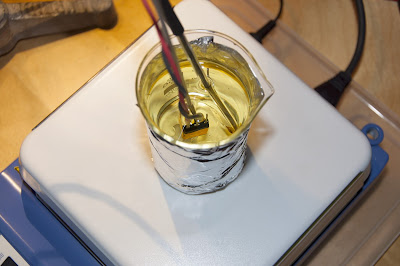## 2016/06/07

### Push it to the limit – measure the true junction temperature to get the most out of MightyWatt

Spoilers!

If you just want to know if you can overload your MightyWatt, the answer is yes. You can run it at 75 W constantly and at about 85 W for short periods.

End of spoilersFig. 1: Experimental setup for measuring forward voltage drop of a FET body diode.

## Introduction

If you want to run a FET at high temperature, which is typical in electronic load applications, it is important not to exceed the maximum junction temperature. In my MightyWatt electronic load, I use IXTH75N10L2 as the main transistor and its maximum allowed junction temperature is 150 °C.

It is not easy to measure the junction temperature without actually having a sensor at the junction. Thermal camera, thermistors and other sensors will always measure less because there will be a temperature gradient betwen the case and the junction. At 70 W, the temperature of the copper tab directly attached to the die can be 20 °C less than the silicon temperature!

Luckily, there is a way of measuring the junction temperature. Sadly, it cannot be done while the load is operating… Because the temperature sensor is the intrinsic body diode of the FET itself. Its temperature coefficient can be measured in laboratory conditions and then its temperature can be correlated to some other temperature sensor, attached to the body of the transistor. In case of MightyWatt, that is a tiny 0402 thermistor placed beneath the transistor.

## Experimental setup, results and discussion (don't mix this in a paper)

### Measurement of the diode temperature coefficient

I have first measured the intrinsic body diode forward drop (VSD) of 10 samples of IXTH75N10L2 at room temperature (about 24 °C) at 1 mA using Keysight 34461A bench-top digital multimeter. Interestingly, they were all the same, at 522 mV. That's good! Seems like the process variation is not very high.

Then, one sample from these 10 was randomly selected and fitted with silicone elastomer insulated cables to the drain and source.

The transistor was then immersed in an oil bath (edible rapeseed oil actually) in a borosilicate beaker with a magnetic stir bar and put on a heated magnetic stirrer (IKA C-MAG HS7) (Fig. 1, 2). The temperature sensor from the stirrer was Kapton-taped to the metal tab of the transistor.Fig. 2: Transistor in an oil bath with two temperature probes: One from the magnetic stirrer/hotplate and a second, external one for verification.

Then, the bath was heated to 60, 70, 80, 90, 100, 110, 120 and 130 °C and when the temperature stabilized, a few seconds worth of experimental values were averaged to get the VSD. The multimeter sampled at about 1000 samples per second.

The VSD turned out to be linearly dependent on the temperature with a slope of -2.454 mV/K (Fig. 3).Fig. 3: Temperature dependence of VSD and ideality factor n (which is only discussed in the "extra math" section so pretend you can't see it).

### Measurement of the diode VSD in MightyWatt

First, I soldered wires to the drain and source of one standard MightyWatt's main transistor. The source was at the common ground potential. Because measuring VSD means connecting the polarity in opposite, the drain was connected to a SPDT relay. The normally closed lead was connected to multimeter negative terminal (diode cathode) via a 0.15-Ohm current sense resistor while the normally open lead was connected to power supply positive terminal.

Triggering of the measurement was done using a comparator circuit, which was driving one transistor that switched the relay and another transistor which triggered the multimeter. When there was current flowing through the load, the comparator would switch the relay to connect the power supply. When the current was interrupted, the relay switched to measure the VSD (Fig. 4). The propagation delay between the current interrupt and the actual trigger signal was measured using an oscilloscope (Rigol DS1052E).Fig. 4: Schematic of the connections for the in-system measurement of VSD.

The load was let to dissipate 75.9 W at 3.004 A and I waited until the temperature reported by a thermistor placed beneath the transistor was stable. This took about 10 minutes from ambient temperature. Then, the current was interrupted by physically removing USB cable that powered MightyWatt, immediately interrupting current flow. At that point, the relay switched and multimeter began to measure 2000 samples at about 1000 samples per second. I am only showing the first 500 ms, since the rest was a monotonic increase.

The VSD quickly increased upon interrupting the current. The trigger propagation delay was about 0.2 ms and thus negligible. There was an 80-ms delay starting from the current interrupt before the sampled data stabilized. The VSD had to be extrapolated from the recorded data to the point where the current was actually interrupted. I used only a phenomenological 5th order polynomial to extrapolate to the beginning (Fig. 5). Thermal fluxes are complicated in such setup…Fig. 5: VSD development with time right after the current was interrupted. First 80 ms had to be extrapolated.

So the VSD was about 260 mV when the current was interrupted, which means the junction temperature was about 132 °C, well below the maximum. By the way, the thermistor reported 101 °C temperature. The ambient temperature was 26 °C.

We can calculate the thermal resistance of the system now: (132 - 26) / 75.9 = 1.4 K/W. While the thermistor thinks it is (101 - 26) / 75.9 = 1 K/W. We should therefore calculate the junction temperature (Tj) from thermistor temperature (Tth) and ambient temperature (Tamb) as Tj = (Tth - Tamb) / 1 × 1.4 + Tamb.

Assuming Tamb = 25 °C and Tj = 150 °C, the thermistor will be reporting about 114 °C at this maximum. MightyWatt will report overheat above 110 °C, which seems to be a correct threshold to allow some leeway.

The maximum dissipated power is then (150 - 25) / 1.4 = 89 W. And at rated 70 W, the junction temperature is about 70 × 1.4 + 25 = 123 °C. But there is one catch: The Arctic Silver AS5 thermal paste (which I use) is rated 130 °C continuously (180 °C for short periods of time). The temperature at the thermal paste is unknown because datasheet only quotes maximum junction-to-case thermal resistance. Using the maximum value, the case temperature at Tj = 150 °C will not be less than 122 °C. But probably it will be higher, possibly exceeding the long-term rating of the thermal paste. So to be perfectly safe, let's assume the Tj to be 130 °C maximum. At this temperature (and 25 °C ambient), the maximum continuous power dissipation is (130 - 25) / 1.4 = 75 W

We should leave some safety margin because the extrapolation might not have been very precise and the Tj could have been higher. But advertising MightyWatt as a 70 W load seems to be honest, if a little conservative. And don't worry overloading it for extra 15 W for short experiments. You will have to change the maximum power in the sketch though.

That's all folks! But if you're interested in some more math, then please, continue reading…

## Digging deeper

### Calculation of VSD

Besides direct measurement, the VSD can be calculated using Shockley diode equation (1), where I is the diode current (1 mA in this case), IS is the reverse bias saturation current, n is the ideality factor, typically between 1 and 2, and VT is the thermal voltage equal to kT/q, where k is the Boltzmann constant, T is the thermodynamic temperature and q is the elementary charge.

(1)     I = IS[exp(VSD/n/VT) - 1]

IS is temperature dependent and is approximately equal to (2), where Eg is the bandgap energy.

(2)     IS = T3exp(-Eg/k/T)

The bandgap energy also depends on temperature and can be calculated from (3), with experimentally determined parameters Eg(0) = 1.166 eV, α = 4.77·10-4 eV/K and β = 636 K.

(3)     Eg(T) = Eg(0) - αT2 / (T + β)

Combining equations 1, 2 and 3 with the experimental data permits the calculation of the only remaining unknown – the ideality factor n – which was calculated to be between 1.061 and 1.175 for the temperature from 60 to 130 °C (Fig. 3).
The value of n indicates that the current was limited mainly by the recombination of minority carriers in the neutral region.

### Junction temperature under load

Thermal fluxes in systems like transistors on an active heatsink are complicated. The heat emerges from a relatively small region made of silicon and is then preferentially conducted via copper tab to the back of the transistor case where a heatsink is attached. The silicon content is very low compared to the lead frame and encapsulant, which contribute to almost the entire heat capacity of the device.

IXYS does not publish information about the material composition of the transistor but a similarity can be assumed with other parts in the same package. International Rectifier publishes Bill of Materials for TO-247 packaged transistors. The main mass (66.2 %) of the transistor is the copper lead frame. The other significant contribution is the epoxy resin, that is composed mainly of a filler – SiO2. These two materials account for over 90 % of the overall mass. The silicon content is a meager 0.6 %. The total weight of the transistor, as reported by International Rectifier, is 5.6 g. The IXYS IXTH75N10L2 was somewhat heavier at 6.4 g.

This permits us to calculate the approximate heat capacity of such transistor. The specific heat capacity at 127 °C of Cu, SiO2 and Si is 398.6, 889.2 and 788.3 J/kg/K respectively. Assuming the balance to 100 % has the same average heat capacity as the two main components, the overall heat capacity of the International Rectifier transistor is approximately (398.6 × 0.003719 + 889.2 × 0.001411)/0.913 = 3.00 J. Scaling this to the IXYS transistor, the heat capacity could be about 3.00 × 6.4/5.6 = 3.43 J.

Assuming the transistor is evenly heated and thermally conductive, the 80 ms delay at 75.9 W of cooled power would cool it by 1.8 K. However, such assumption is never true because the materials have different thermal conductivity and the heat distribution is uneven, with the maximum at the junction. Besides that, the silicon part has very low heat capacity because it is very small and the thermal path is mainly through the copper to the heatsink. There is a large temperature gradient from the junction to case at 75.9 W. According to the datasheet, the junction-to-case thermal resistance is up to 0.31 K/W, which creates a gradient of 23.5 K at 75.9 W. The extrapolation of junction temperature, even to a time only 80 ms before experimental points, is not trivial and in this study was done by simply fitting the VSD vs. temperature curve with a 5th order polynomial that accurately tracked the experimental data (Fig. 5).

Well, if you read all of this, you really pushed it to the limit. Go ahead, you deserve it!